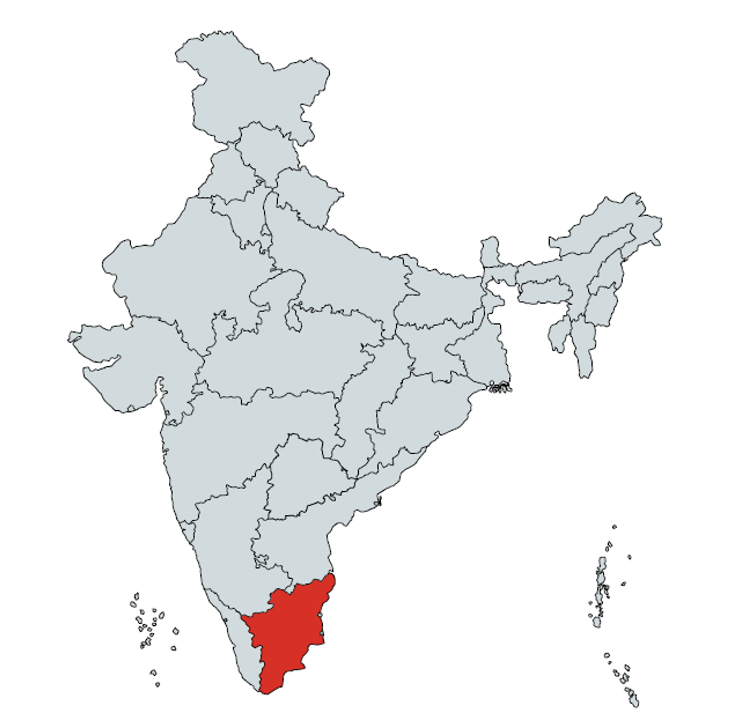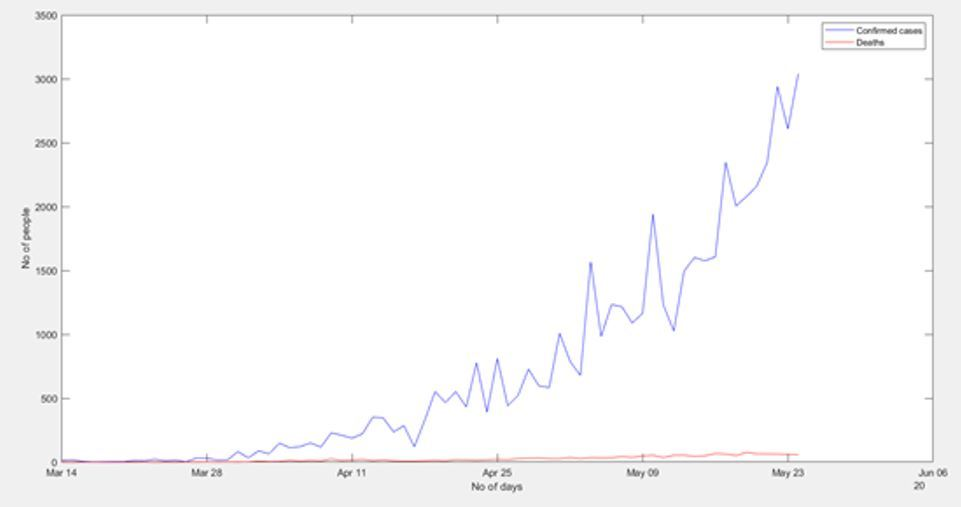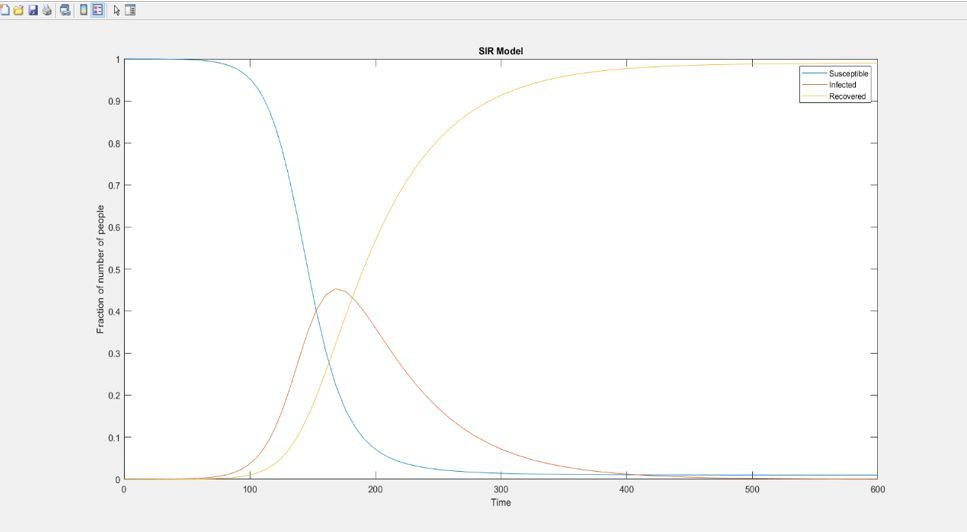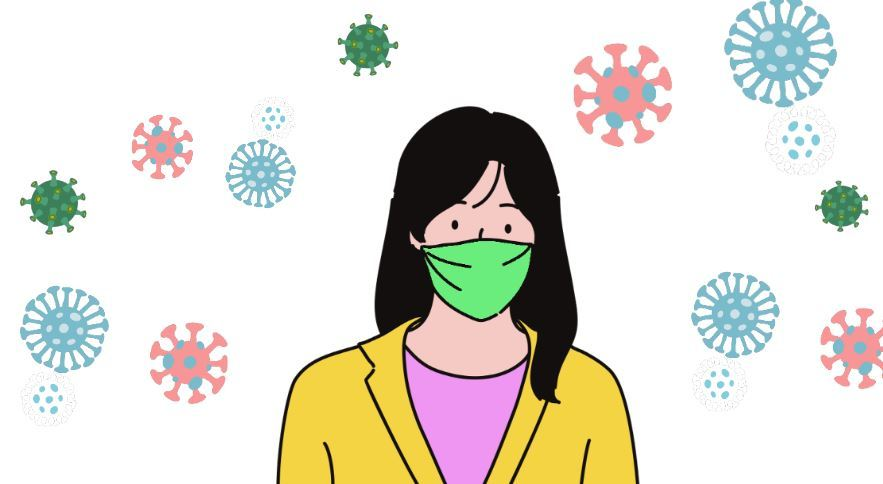# COVID-19 SIR Modelling for Tamil Nadu, India

June 13, 2020

By  Roshini

###### Move quickly from one page to another within COVID-19 Series from MATLAB Helper:

In this blog, we are going to show the pandemic analysis of another state of India, which is Tamil Nadu (TN).We are starting this approach by collecting the data. In this case, the data is not collected from an online source, but an excel sheet is used for the same. So using 'readtable' is possible to read the data and collect some part of it as well. You can download the CSV file from here: http://api.covid19india.org/csv/latest/state_wise_daily.csv.

%%
%Reading the data from .csv file
time=(table2array(time));
time=(time(1:3:end,1))';
TN=table2array(TN);
%%
%Initializing the variables
N=6.79e7;
I=(TN(1:3:end,1))';
R=(TN(2:3:end,1))';
D=(TN(3:3:end,1))';
S=N-I-D;

Here, data is pre-processed by using table2array function and population (N), susceptible (S), infected (I) and recovered (R) individuals are computed. For this time, the data into excel sheet was chosen to be related to Tamil Nadu population.

## Estimating ???? and ????

These parameters are computed according to equations  and  shown in the SIR epidemic model section in our earlier blog post.

s=S/N;
i=I/N;
r=R/N;
%%
%Finding b and k
b=-1*([0 diff(s)]./(s.*i));
b(isnan(b)) = [];
b(isinf(b)) = [];
b=abs(mean(b))
k=([0 diff(r)]./i);
k(isnan(k)) = [];
k(isinf(k)) = [];
k=abs(mean(k))

Finally, ???? and ???? are computed as the mean value of their resulting vectors. Additionally, another consideration was taken for cases with ???? and ???? as infinity or Nan.

b =

0.0863

k =

0.0186

## Building and Plotting SIR model

A function called ypsir contains the differential equations, this is shown below:

function ypsir =ypsir(t,y)
a = 0.0863 ;%b value obtained from main code
b = 0.0186 ;%k value obtained from main code
ypsir(1) =-a*y(1)*y(2);
ypsir(2) = a*y(1)*y(2)-b*y(2);
ypsir(3) = b*y(2);
ypsir = [ypsir(1) ypsir(2) ypsir(3)]';

Now setting initial conditions and using ode45 and plot function, we can plot the model response.

%%
%Solving the equations
to = 0;
tf =600;
yo = [s(end) i(end) r(end)];
[t y] = ode45('ypsir',[to tf],yo);
%%
%Plotting the current data
figure();
plot(time,I,'b',time,D,'r');
xlabel('No of days');
ylabel('No of people');
legend('Confirmed cases','Deaths');
%%
%Plotting the prediction
figure()
plot(t,y(:,1),t,y(:,2),t,y(:,3))
title('SIR Model');
xlabel('Time')
ylabel('Fraction of number of people')
legend('Susceptible', 'Infected', 'Recovered')

## Results# Conclusion

We have used data untill 31st May 2020 for all these analysis. We have seen some approaches to modelling diseases such as COVID-19 through MATLAB. We have found essential characteristics within this process. We have done Data Analysis and Data Extraction and included a reliable source for the COVID-19 data. We have used preprocessing to extract a subset of the collected data and organise the data accurately. We have done Data Modelling with model construction, i.e. finding the best model that fits the COVID-19 pandemic. We have done the calculation of model parameters using real collected data and finally done the Data Visualisation. All these steps were essential, and luckily, MATLAB has so many functions and tool to use in the given scenario.

Now summing our analysis and coming to a definite conclusion, India's COVID-19 case tally climbed from 100 to one lakh in just 64 days as per the data sourced from the Union Ministry of Health & Family Welfare (MoHFW). On comparing this with global data, sourced from Worldometers, it has been found out that India's COVID-19 growth rate is more than double the number of days as compared to Colombia.

### Important Note

Our COVID-19 codes and analysis have been made for educational and research purpose. We have shown different approaches of Pandemic Modelling for each state and the accuracy of result is not guaranteed for real-life situation. We wish for early end of this pandemic. Now it's in our hands; we need to take our responsibilities & take proper precautions.

Now let's have a quick view of the precautions.

#### Stay Home for non-essential activities!Avoid going to crowded areas. Work From Home and Be A Super HeroMaintain distance while conversing with someone. Avoid touching your face frequentlyWash your hand for 20 seconds.  KEEP A SANTISER ALWAYS with you, wherever you go.While sneezing or coughing, always cover your mouth with a handkerchief or with your arm.

#### Home QuarantineIf you have been facing the symptoms of Cold and Cough or the one's shown with COVID-19, then self-isolate yourself with home quarantine for 14 days.  So stay home stay safe; this is the best way you can contribute to the betterment of the nation.

#### Roshini

An Electronics and Communication engineering student. Passionate about learning and sharing with others.

•Nikita Mahoviya says:

The code and explanation are short and crisp, which fulfills the need for basic understanding!!

#### Connect with MATLAB Helper ®

Use Website Chat or WhatsApp at +91-8104622179

Watch our Latest Video

Join a Training Module